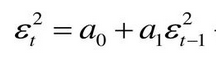# Can we show this in a picture/scatterplot?

Can anyone supply a visual to show what the explanation is describing? My learning curve for time series models has taken longer than anticipated.

I think no scatter plot needed.

Long story short, ARCH model is applied to the error terms calculated from an original AR, MA or ARMA model. The ARCH model is used to find or discard the presence of heteroskedasticity in the original model. That heteroskedasticity is bad because it would imply the original model is not well specified (errors of the original model don’t depict a normal distribution centered at 0).

So, in an ARCH(1) model:if a1 is statistically significant different than 0, then the error terms depict heteroskedasticity, therefore the variance of the error terms ARE NOT constant equal to a0. Hence, we are able to estimate the variance of the error term, otherwise the there would not exist variance of error term because it was constant (a0).

This is like, if you proof something exists, then you can’t deny its existence. If a1 > 0, then variance of errors >0 (it exists, we can estimate it).